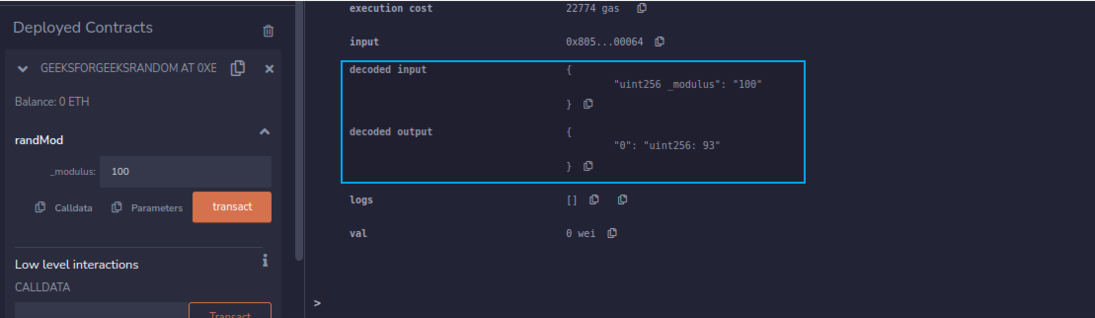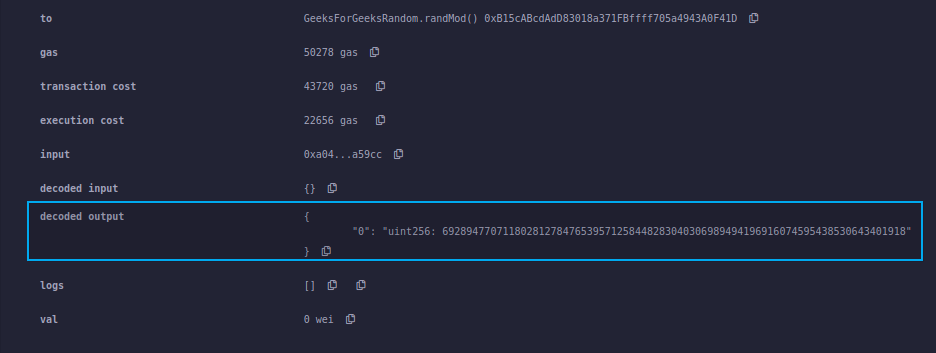Open in App
Not now

# Random Number Generator in Solidity using keccak256

• Difficulty Level : Medium
• Last Updated : 24 Feb, 2023

Random numbers are numbers that occur in a sequence with the following two mandatory conditions:

1. The values are uniformly distributed over a set interval.
2. One cannot predict future values based on past outputs.

Example: In the below example, we have created a contract with a function that will generate a random number in a specific range. Below is the step by step description of the entire process to be followed.

Step 1: Take the instance of block.timestamp, the msg.sender, and an incrementing nonce.

Step 2: “pack” the inputs and use keccak256() to convert into 256-bit hash.

Step 3: Convert that hash to an uint, and then use  modulus (%100) to take only the last 2 digits. This will give us a totally random number between 0 and 99.

## Solidity

 `// SPDX-License-Identifier: GPL-3.0``pragma solidity >=0.4.22 <0.9.0; ``/// @title A contract for demonstrating random number generation in specific range``/// @author Jitendra Kumar``/// @notice For now, this contract just show how to generate a random number in specific range using keccak256``contract` `GeeksForGeeksRandom``{``    ``// Initializing the state variable``    ``uint randNonce = 0;` `    ``// Defining a function to generate``    ``// a random number``    ``function randMod(uint _modulus) external returns(uint)``    ``{``        ``// increase nonce``        ``randNonce++;``        ``return` `uint(keccak256(abi.encodePacked(block.timestamp,msg.sender,randNonce))) % _modulus;``    ``}``}`

Output:Remix Console Output

Step 4: If you do not want to get the random number in specific range than use the below smart contract which returns a large random number.

## Solidity

 `// SPDX-License-Identifier: GPL-3.0``pragma solidity >=0.4.22 <0.9.0; ``/// @title A contract for demonstrate random number generation``/// @author Jitendra Kumar``/// @notice For now, this contract just show how to generate a random number in Solidity using keccak256``contract` `GeeksForGeeksRandom``{``    ``// Initializing the state variable``    ``uint randNonce = 0;` `    ``// Defining a function to generate``    ``// a random number``    ``function randMod() external returns(uint)``    ``{``        ``// increase nonce``        ``randNonce++;``        ``return` `uint(keccak256(abi.encodePacked(block.timestamp,msg.sender,randNonce)));``    ``}``}`

Output:Remix Console Output

Note: The alias “now” for block.timestamp was removed in the version 0.7.0 ,  you can use block.timestamp in place of now  for the latest  solidity version.

Possible attacks with this approach:

In Ethereum, all nodes are trying to solve the problem and verify the transaction. Once a node verifies it, it broadcasts it to the network.

Suppose we create a DApp where we flip a coin where the head is the winning side. We use the above function to predict heads or tails. If I were running a node, I could publish a transaction only to my own node and not share it. I will run the randMod function or the coin flip function until I win, and will only share the transaction after I have won.

One way to solve this would be to use an oracle to access a random number function from outside the Ethereum blockchain. There are other cryptographic algorithms and third party functions that can be utilized, but they are not safe or should be audited.

My Personal Notes arrow_drop_up